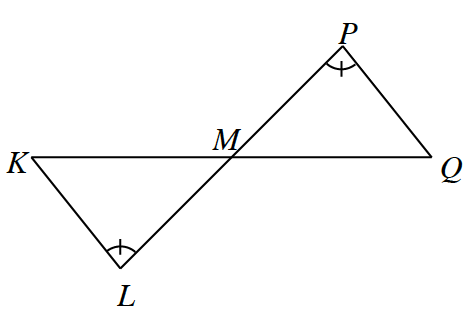### Home > INT2 > Chapter 10 > Lesson 10.2.1 > Problem10-60

10-60.

Examine the diagram at right. If point $M$ is the midpoint of $KQ$ and if $∠P ≅ ∠L$, prove that $KL ≅ QP$. Write your proof in flowchart format.

Recall that vertical angles are congruent.

What is the definition of a midpoint?